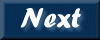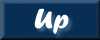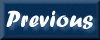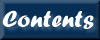Next: 10. References Up: XRONOS User's Guide Previous: 8. QDP/PLT   Contents

Subsections

# 9. Common Parameters

The primary XRONOS tasks, autocor, crosscor, efold, efsearch, listdata, lcmath, lcstats, lcurve, powspec, and timeskew, share common parameters:

The following abbreviations are used to indicate which XRONOS tasks use a common parameter:

• AC - autocor
• CC - crosscor
• EF - efold
• ES - efsearch
• LC - lcurve
• PS - powspec
• TS - timeskew
• LD - listdata
• LS - lcstats
• LM - lcmath
• BC - earth2sun (barycenter correction)
• RF - rbf2fits

# 9.1 General Execution

## 9.1.1 tchat

(Integer parameter; hidden; d/f=5; range 0-10)

Set terminal chattiness: (0-4) only little information is output in running XRONOS applications; (5) default chattiness; (6-7) more details on input files, windows, intervals statistics, etc.; (8) mostly for debugging purposes.

## 9.1.2 lchat

(Integer parameter; hidden; d/f=0; range 0-10)

Set log file chattiness: the default (=0) is not to write any log file; for all other values information is written to the log file, with a chattiness which is specified by the value of this parameter

## 9.1.3 logname

(Character parameter; hidden; d/f=xronos.log)

Specify the log filename, which records all interaction with a XRONOS task and results from the analysis which are normally output only to the terminal.

Tasks: AC CC EF ES LC PS TS LD LS BC

## 9.1.4 dpath

(Character parameter; hidden; d/f=XRDEFAULTS)

Specify the path to the Xronos "defaults" directory, which contains the default .pco file (used for plotting) and the default windows file default_win.wi. Ordinarily, the user may leave this parameter set to the string XRDEFAULTS, which causes Xronos to use the environment variable XRDEFAULTS to locate these files. XRDEFAULTS is set when the lhea-init script is sourced to point to the appropriate directory for the current distribution of Xronos (\$LHEASOFT/xrdefaults). If the user wishes to modify these files, he or she may make and edit copies, and change the XRDEFAULTS environmental variable appropriately, but the original files should not be changed.

Tasks: AC CC EF ES LC PS TS LD LS

# 9.2 Output File

## 9.2.1 clobber

(Logical parameter; hidden; d/f=yes)

By default, if the output file has the same name as an existing file, it is overwritten. Set clobber=no to cause a warning to be printed, leaving the original file intact.

Tasks: AC CC EF ES LC PS TS

## 9.2.2 outfile

(Character parameter; queried; d/f= default)

Name of the output file. If only the root is given, the program adds an extension of 3 characters, .fXX, where f stands for FITS and XX is a suffix which identifies the program that creates the output. The XX values are : ac (autocor), cc (crosscor), ef (efold), es (efsearch), lc (lcurve), ps (powspec), ts (timeskew). Typing default forces the program to generate a default value for the output filename (see outfileroot parameter). By typing blank characters at the prompt no output file will be created.

Note, although lcmath, earth2sun, and rbf2fits all contain an outfile parameter, it doesn't behave exactly as described above. See the individual tasks for the correct outfile description.

Tasks: AC CC EF ES LC PS TS

## 9.2.3 outfileroot

(Character parameter; hidden; d/f= default)

This parameter used in conjunction with outfile generates a default output filename. If the value of outfileroot is default and outfile is default, the output filename will have the same root name of the first input file of the fits series with a .fXX extension (see outfile for XX values).

Tasks: AC CC EF ES LC PS TS

## 9.2.4 outfiletype

(Integer parameter; hidden; d/f= 2)

Define the FITS structure for the output file. Two types are available. The first type (outfiletype=1) has 1 interval of results per FITS table row. The output file will have only one extension. Each row of the results column contains an array of values with a size equal to the number of points per interval (or frame) and a number of rows equal to the valid number of intervals analysed. The second type (outfiletype=2) has 1 interval of results per FITS extension. The output file will have as many extension as the number of intervals (or frames) analysed. The column containing the results has a single element value in each row and the number of rows is equal to the number of points in the interval. The default value of outfiletype is 2 (one interval per FITS extension).

Tasks: AC CC EF ES LC PS TS

# 9.3 Plotting

## 9.3.1 plot

(Logical parameter; queried; d/f=yes)

Specify whether the results of the analysis are plotted via PLT. NOTE: After plotting the results the XRONOS task is left in the interactive plotting/fitting mode (PLTprompt) use command exit to return and finish the XRONOS task. If the plot parameter is set to "no", the other queries regarding the plotting are disabled (plotdev, plotfile).

Tasks: AC CC EF ES LC PS TS

## 9.3.2 plotdev

(Character parameter; queried; d/f=/XW)

Specify the plot device. The most common plot devices are /xw (X window), /tek (tektronix), /vt (vt125), /ps (PostScript). Setting plotdev as "?", forces the program before plotting to list the available plot devices to the terminal and to prompt for this parameter again.

Tasks: AC CC EF ES LC PS TS

## 9.3.3 plotfile

(Character parameter; hidden; d/f=-)

Name of the Plt COmmands file. For each task an appropriate default Plt COmmand file is automatically loaded by the program when the parameter plotfile is "-". The user can insert additional commands using a custom .pco file at this level (or within the PLTprompt). The user commands are appended to the default command file and therefore executed last. The default .pco files are stored in the directory defined by the parameter dpath.

Tasks: AC CC EF ES LC PS TS

# 9.4 Windows

## 9.4.1 window

(Character parameter; queried; d/f=-)

Filename of the xronos window file. The window file is an ASCII file and by default a standard window file is used, where only exposure windows are set. To modify the standard file or create a new file use the script xronwin.

Tasks: AC CC EF ES LC PS TS LD LS

## 9.4.2 spwinbefore

(Real parameter; hidden; d/f=0.; range 0 -)

Special newbin window : number of seconds before. If a value0 is used , e.g. 10.0, then all the newbins within 10.0 seconds before a newbin rejected by an intensity window will also be rejected. The default (=0) is not to apply this type of special newbin window.

Tasks: AC CC EF ES LC PS TS LS

## 9.4.3 spwinafter

(Real parameter; hidden; d/f=0.; range 0 -)

Special newbin window : number of seconds after. If a value0 is used , e.g. 10.0, then all the newbins within 10.0 seconds after a newbin rejected by an intensity window will also be rejected. The default (=0) is not to apply this type of special newbin window.

Tasks: AC CC EF ES LC PS TS LS

# 9.5 Rebinning

## 9.5.1 dtnb

(Real parameter; queried)

The duration in seconds of the NEWBIN time. For binned input files the NEWBIN duration can not be shorter than the longest bin duration in the input file. In autocor, crosscor, powspec, and timeskew the NEWBIN time must be an integer multiple of the minimum newbin time. The task internally calculates (and prints on the screen) a default value such that a single interval is produced with a fixed number of newbins (typically between 128 and 4096 depending on the task and on the time interval length see also nbdf parameter). Typing INDEF forces the task to use as NEWBIN time the value calculated by the program. NOTE: By pressing "Return" the task will use as NEWBIN time the value found in the parameter file used in a previous run.

Tasks: AC CC LC PS TS LS

## 9.5.2 nbint

(Integer parameter; queried)

The number of newbins per interval used in the analysis. The nbint together with the NEWBIN duration (dtnb) determines the length in time of an interval and therefore the total number of intervals within the start and stop time over which the analysis will be carried out. Typing INDEF forces the task to use the default value (see parameter nbdf). NOTE: By pressing "Return" nbint is set to the value found in the parameter file used in a previous run. In autocor, crosscor, and powspec, if fast=yes (the default), nbint must be a power of 2 in order to use an FFT algorithm.

Tasks: AC CC EF ES LC PS TS LS

## 9.5.3 nintfm

(Integer parameter; queried)

The results of the analysis from individual intervals can be averaged to produce a frame. The nintfm parameter is used to specify the number of intervals averaged in a frame. Typing INDEF forces the task to use the default value (all intervals will be use in one frame). NOTE: By pressing "Return" nintfm is set to the value found in the parameter file used in a previous run.

Tasks: AC CC LC PS TS

## 9.5.4 nphase

(Integer parameter; queried)

Number of phases in the folded light curve(s). Typing INDEF forces the task to use the default value (see parameter nbdf). NOTE: By pressing "Return" nphase is set to the value found in the parameter file used in a previous run.

## 9.5.5 nbdf

(Integer parameter; hidden)

Specify an internal default value for the number of newbins per Interval. This value is used to calculate the default newbin integration time to have one interval with nbdf points. For efold and efsearch, however, the value is in phasebins per period. Different nbdf values have been set for different XRONOS tasks. IMPORTANT NOTE: With caution this parameter can be changed by the user.

Tasks: AC CC EF ES LC PS TS LD LS

## 9.5.6 rebin

(Integer parameter; queried; d/f=0)

The results of the analysis of individual frames can be rebinned either with a constant rebinning factor or as a geometrical series (to produce nearly equispaced bins in a logX representation). The default value (0) is not to rebin the results. Values greater than 1 indicate a constant rebinning factor. Geometrical series rebinning is obtained by entering a value less than -1, whose modulus represents the step of the geometrical series (Values between -1.4 and -1.2 are adequate in most cases).

# 9.6 Trend Removal

## 9.6.1 itre

(Integer parameter; hidden; d/f=0; values=0,1,2,3,4)

A polynomial trend, up to 4th-order, can be removed from input time series. Setting the parameter itre equal to 1, 2, 3 or 4 removes a 1st, 2nd, 3rd or 4th-order polynomial trend, respectively. The trend is determined separately for each interval of each series by using a least-square technique. The value 0 does not cause the removal of any trend from the input series and is the default value.

Tasks: AC CC LC PS TS LS

## 9.6.2 itremo

(Integer parameter; hidden; d/f=1; values=1,2,3)

Specify how the trend removal is applied to the data (available only if itre is higher than 0). The trend can be subtracted from the time series (itremo=1), or the time series can be divided by the trend (itremo=2), or the time series can be replaced with the trend (itremo=3). By default the trend is subtracted (value set to 1).

Tasks: AC CC LC PS TS LS

# 9.7 Data Scaling

## 9.7.1 rescale

(Real parameter; hidden; d/f=1.)

Rescaling factor applied to result variables and errors. The rescaling is applied just before writing the output file (this to avoid affecting the statistical variables for the frame).

Tasks: AC CC EF ES LC PS TS LS

## 9.7.2 offset

(Real parameter; hidden; d/f=0.)

Additive constant summed to result variables. Result error bars are left unchanged. The additive constant is added just before writing the output file (this is to avoid affecting the statistical variables for the frame). Note that if a rescaling factor is also specified (different from 1), then the results are first multiplied by the rescaling factor.

Tasks: AC CC EF ES LC PS TS LS

# 9.8 Other

## 9.8.1 gapfill

(Integer parameter; hidden; d/f=0; range 0-)

Replace gaps in input series with running mean. If =0 (the default) data gaps are not filled. If =n newbin data gaps in input series are filled in with running mean values calculated over n newbins. Note that a gap newbin is filled in only if the corresponding running mean is calculated over at least n/4 points (this means that in order to bridge a gap of m newbins n must be1.35m).

Tasks: AC CC LC PS TS LS

## 9.8.2 forcestart

(Logical parameter; hidden; d/f=no)

If =yes the first interval will be forced to start at the time of the first time window otherwise (=no default) the center time of the first qualified newbin is used as the start time.

Tasks: AC CC EF ES LC PS TS LS

## 9.8.3 exposure

(Logical parameter; hidden; d/f=no)

If =yes the exposure profile(s) (i.e. newbin values are set =1, gaps and rejected newbins are set =0) is/are analysed (instead of the input series).

Tasks: AC CC EF ES LC PS TS LSNext: 10. References Up: XRONOS User's Guide Previous: 8. QDP/PLT   Contents